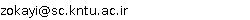••••••••## “Ali Reza Zokayi”

Tel:  +98 21 2853884
Fax:  +98 21 2853884
Email:### IPM Positions

Non Resident Researcher (non-resident), School of Mathematics
(2002 - 2003 )

### Non IPM Affiliations

Assistant Professor of Khajeh Nasir Toosi Technical University

Finite Groups

### Research Activities

Characterization of finite groups through their element orders is one of the most important problem in group theory after the full classification of finite simple groups, which was completed in 1981. This problem was first introduced by Prof. W.J. Shi. Soon later W.J. Shi investigated the finite groups in [SY] whose element orders are of prime order except the identity element and he got the interesting result: The alternating group A5 can be characterized only by its element orders. At this time many researchers such as W.J. Shi, H. Deng, V.D. Mazurov, A. Zavarnitsine, M.R. Darafsheh, A.R. Moghaddamfar and myself, M.S. Lucido, R. Brandl, C.Y. Tang, S. Lipschutz, J. An etc, are working on this problem.

Let G be a finite group. Denote by pe(G) the set of all orders of elements in G. Obviously pe(G) is a subset of the set N of natural numbers, and it is closed and partially ordered under divisibility. We sometimes let m(G) to be the set of maximal elements of (pe(G), |).

Let ? ? W ? N. Now we ask the following questions:

(1) Is there a finite group G with pe(G)=W ?

(2) If the answer is affirmative then how many non-isomorphic groups exist with the above set of element orders?

Certainly, W must have the following properties: (a) 1 ? W and (b) W must be closed and partially ordered with respect to divisibility. The conditions (a) and (b) are necessary but not sufficient, for example there does not exist any group G with pe(G)=W = {1, 2, 3, 4, 5, 6, 7, 8, 9 }. In fact R. Brandl and W.J. Shi in [BS] have classified all finite groups whose element orders are consecutive integers and in this paper they have shown that if pe(G)={1, 2, 3, ..., n }, for some group G, then n ? 8. Therefore, we consider a concrete group, say M, and set pe(M)=W. Now it is evident that the answer of question (1) for such W is positive. So we try to answer the question (2). Let h(W) be the number of non-isomorphic classes of finite groups G such that pe(G)=W. Given a group G, it is obvious that h(pe(G)) ? 1. Now, we make the following definitions:

A group G is called non-distinguishable if h(pe(G))=?.

A group G is called k-distinguishable if h(pe(G))=k < ?.
Moreover, 1-distinguishable groups are simply called characterizable.

Professor W.J. Shi has recently conjectured that h(pe(G)) ? {1, 2, ?}, for any group G.

It is well known that:
?

 Aut(PSL(2,pn))=PGL(2,pn): Zn .

When n=1, we have Aut(PSL(2,p))=PGL(2,p) , and hence
?

 m(PGL(2,p))=m(Aut(PSL(2,p)))={p-1,p,p+1}.

In this research, we consider almost simple groups PGL2(p), with 5 ? p < 100, and we show that h(pe(PGL2(p))) ? {1, ?}. In fact we will prove the following theorem:
Main Theorem. Let p be a prime number and 5 ? p < 100. Then h( pe(PGL2(p))) ? { 1,?} .

Proof of such Theorems, strengthen the Shi's conjecture.
References

{[BS ] R. Brandl and W.J. Shi, Finite groups whose element orders are consecutive integers, J. Algebra, 143 (2) (1991), 388-400.
{[SY ] W.J. Shi and W.Z. Yang, A new characterization of A5 and the finite groups in which every non-identity element has prime order, J. of Southwest China Teachers College (in Chinese), 1 (1984), 36-40.

### Present Research Project at IPM

Characterization of finite groups by their element orders

### Related Papers

 1. M. R. Darafsheh, A. R. Moghaddamfar and A. R. Zokayi The characterization of PGL(2, p) for some p by their element ordersInt. Math. Forum 1 (2006), 1825-1831  [abstract]
 2. A characterization of finite simple groups by the degrees of vertices of their prime graphsAlgebra Colloq. 12 (2005), 431-442  [abstract]
 [Back]
scroll left or right